# Canonical Factors for Hybrid Neural Fields

@ ICCV 2023
1UC Berkeley
2TTIC

TLDR Factored feature volumes have an axis-alignment bias.
This can be addressed via a learned set of transforms!## Overview

We study state-of-the-art neural field architectures that rely on factored feature volumes, by (1) analyzing factored grids in 2D to characterize undesirable biases for axis-aligned signals, and (2) using the resulting insights to propose TILTED, a family of hybrid neural field architectures that removes these biases.

## Qualitative Results

We explore how recovering canonical factors via a learned set of transformations can improve factorization-based representations with only a handful of extra parameters.

For signed distance fields, this reduces floating artifacts:For radiance fields, it improves visual fidelity: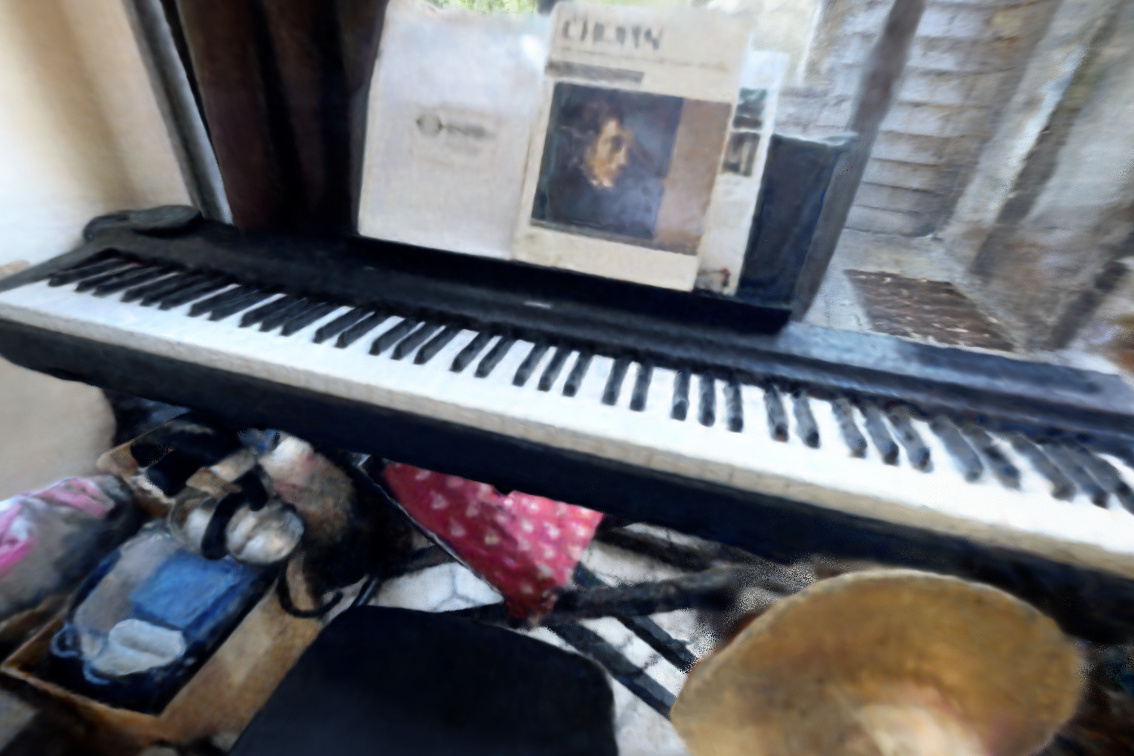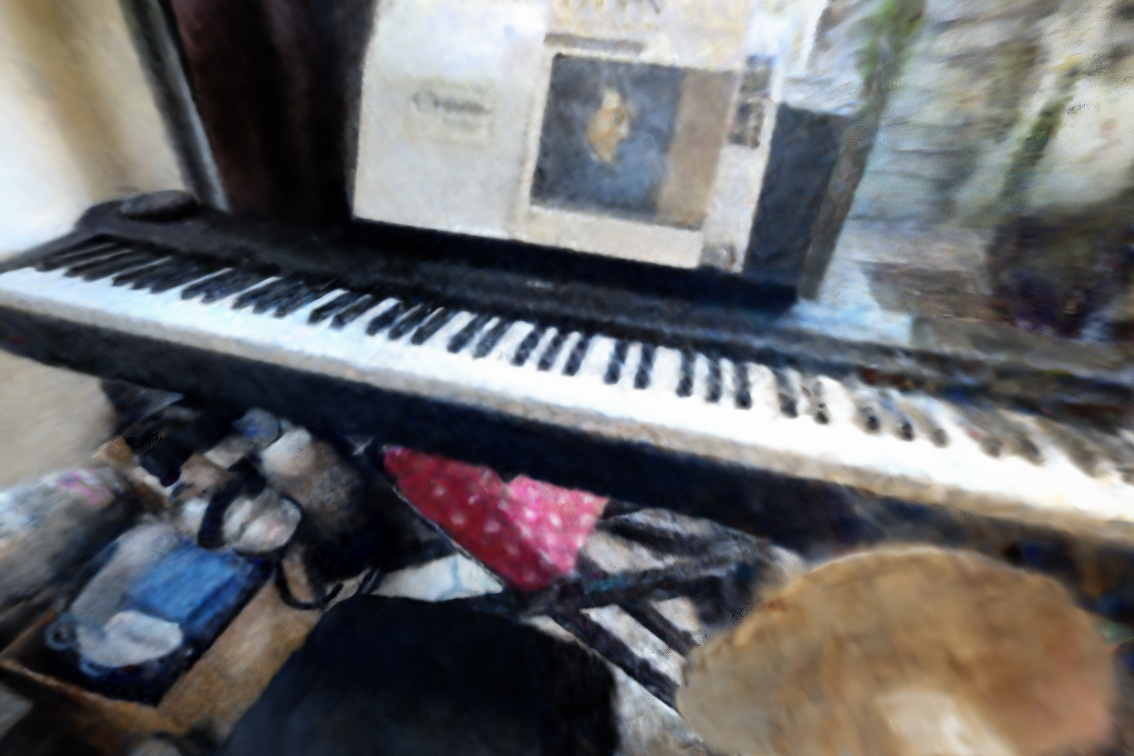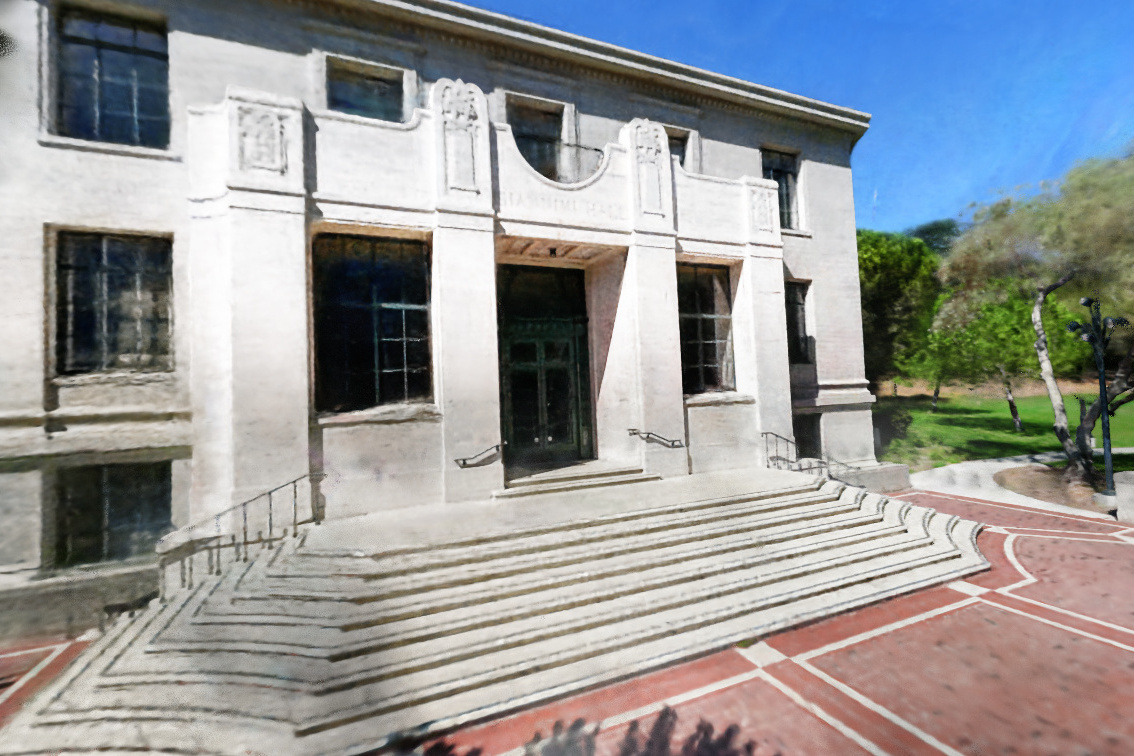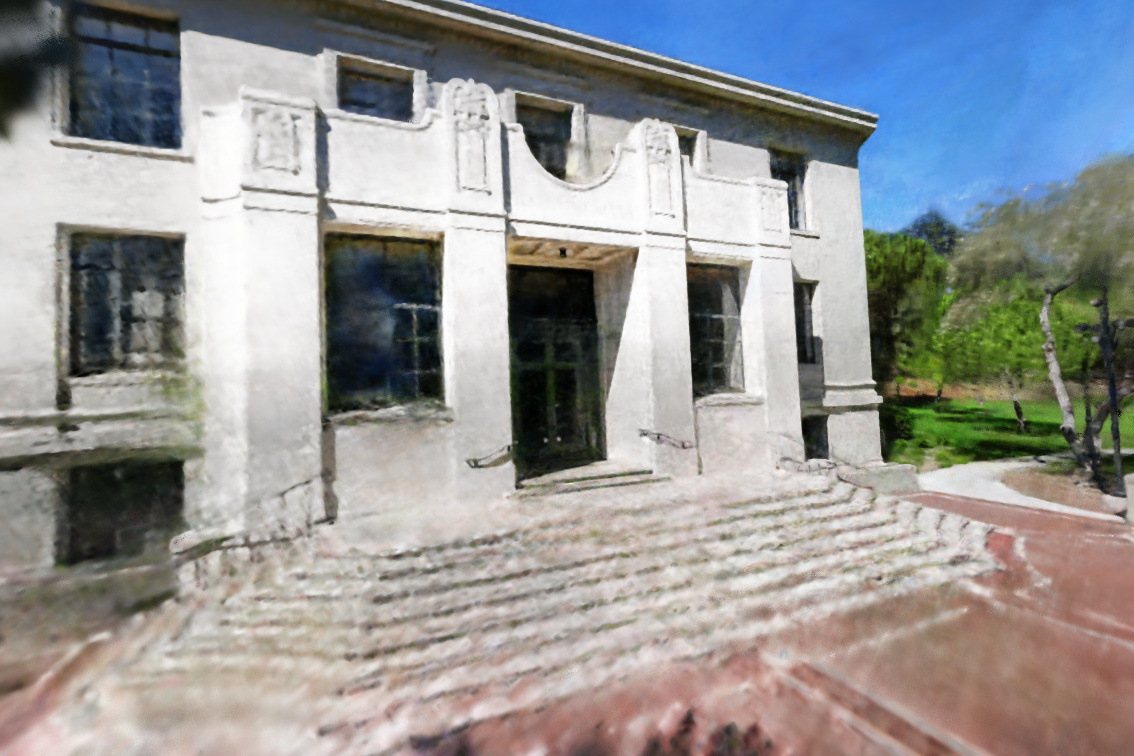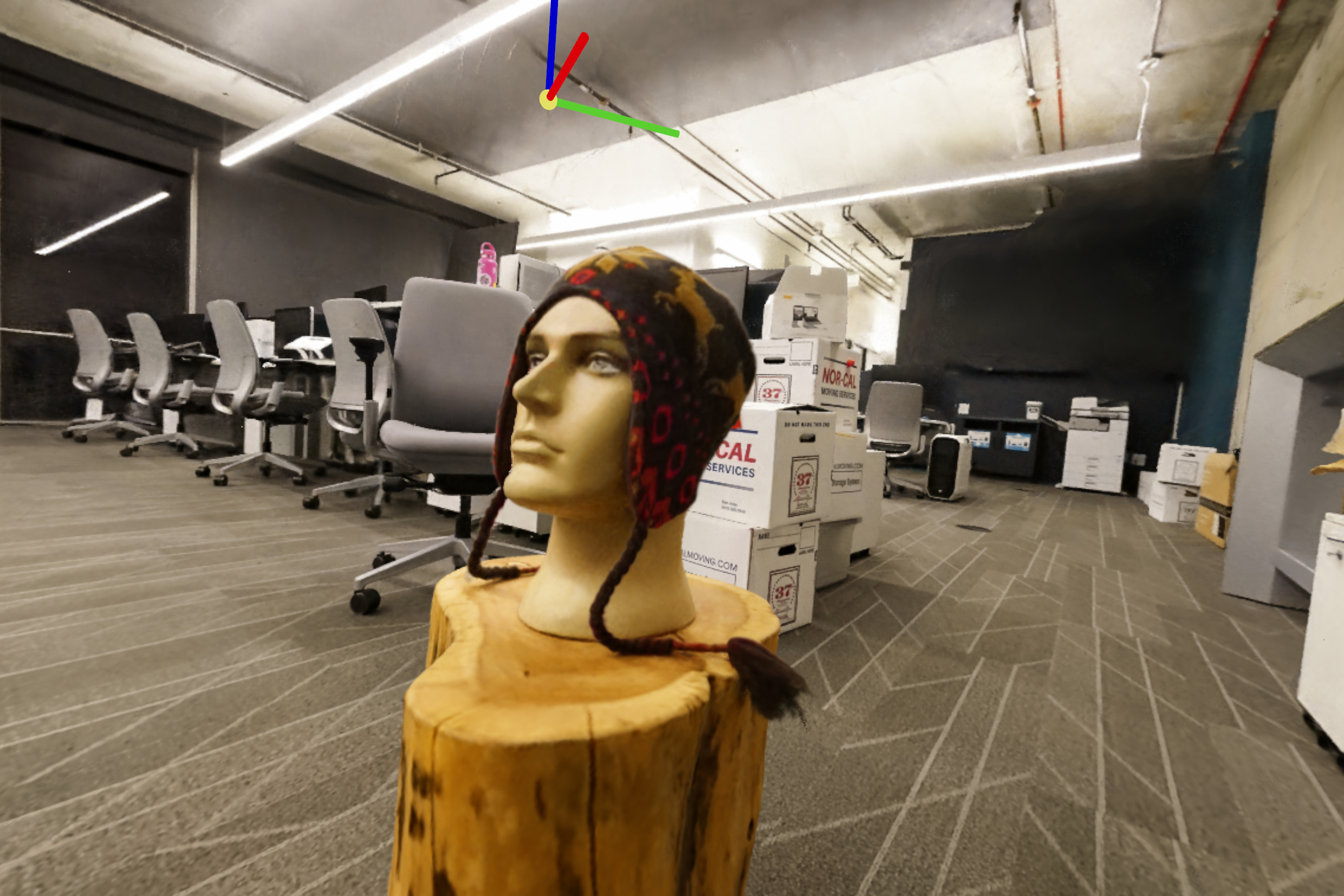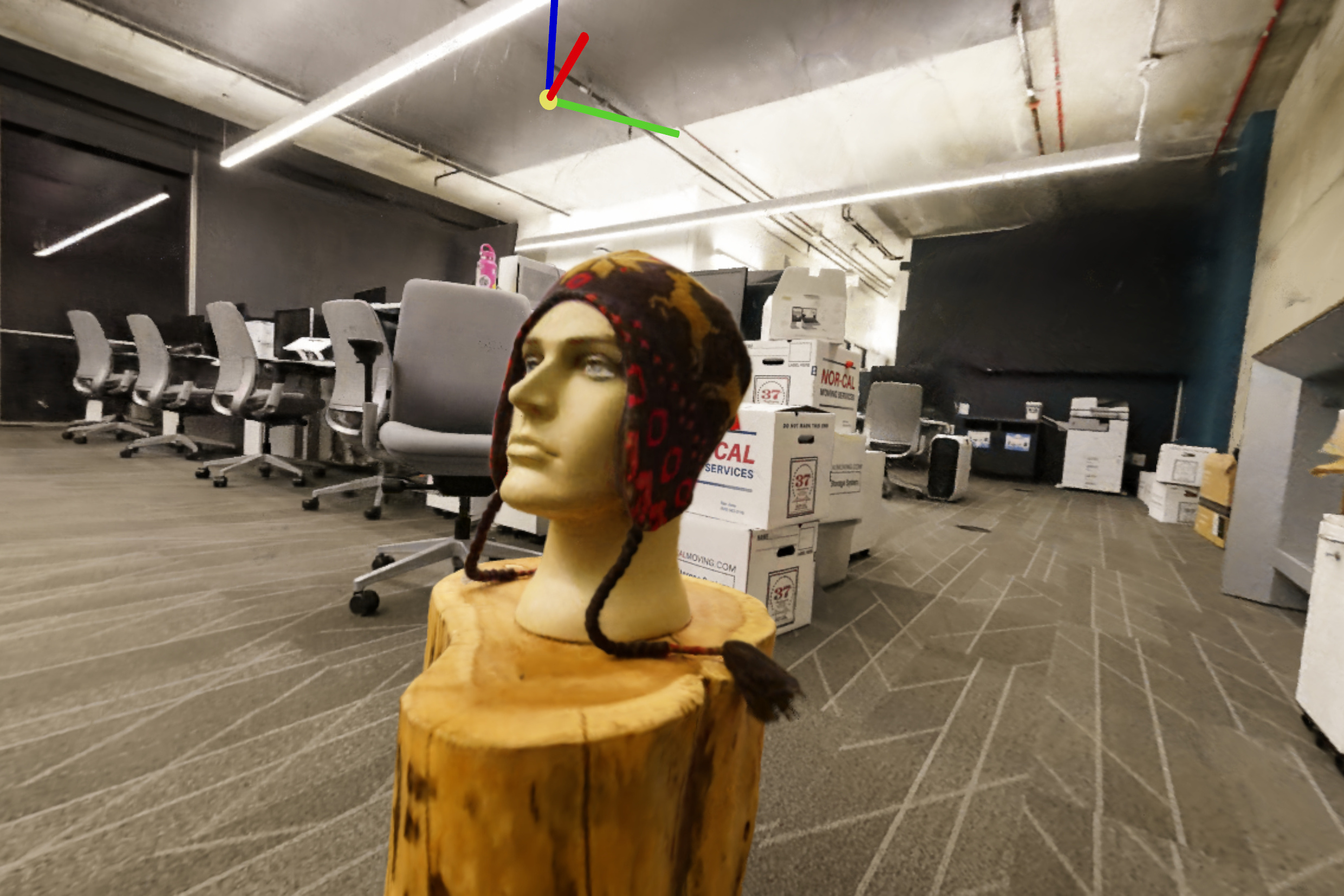From the norms of interpolated features, we observe that canonicalized factors interpretably align themself to scene geometry: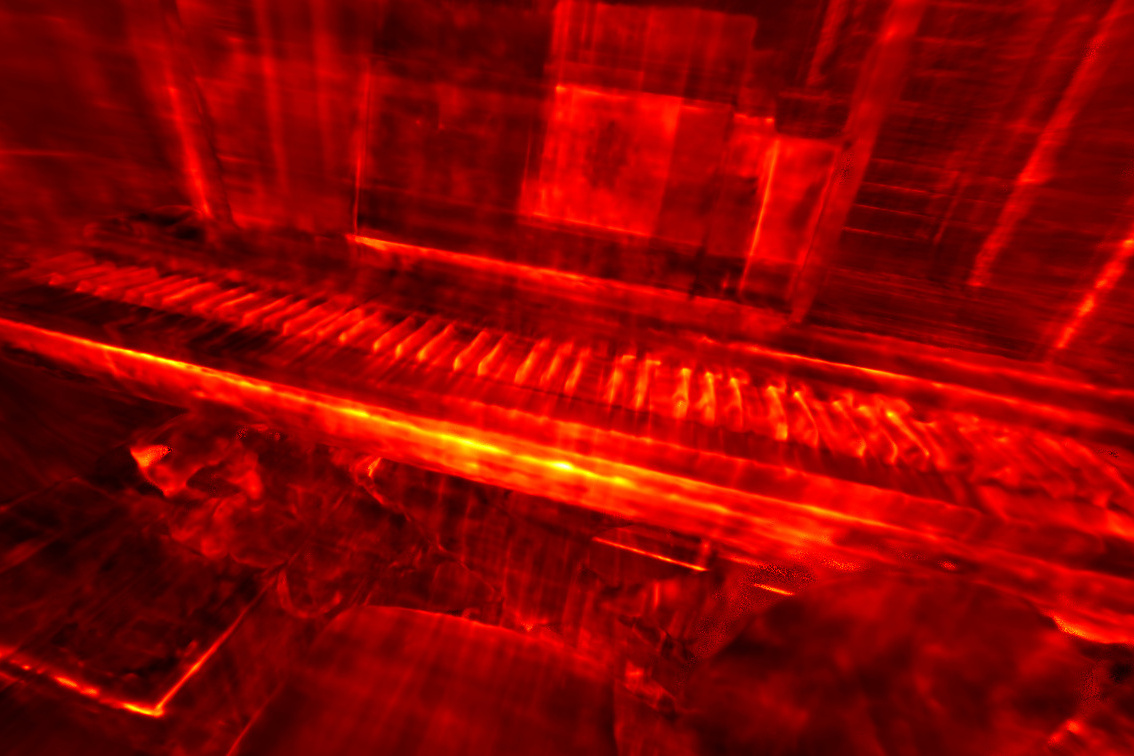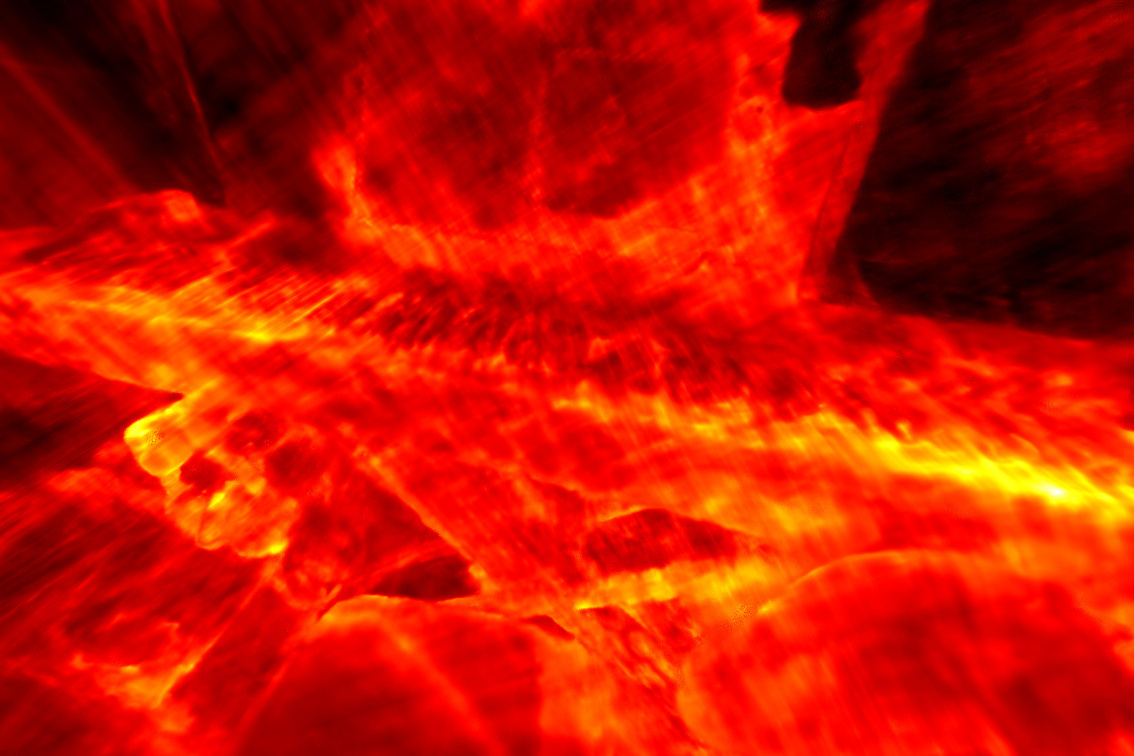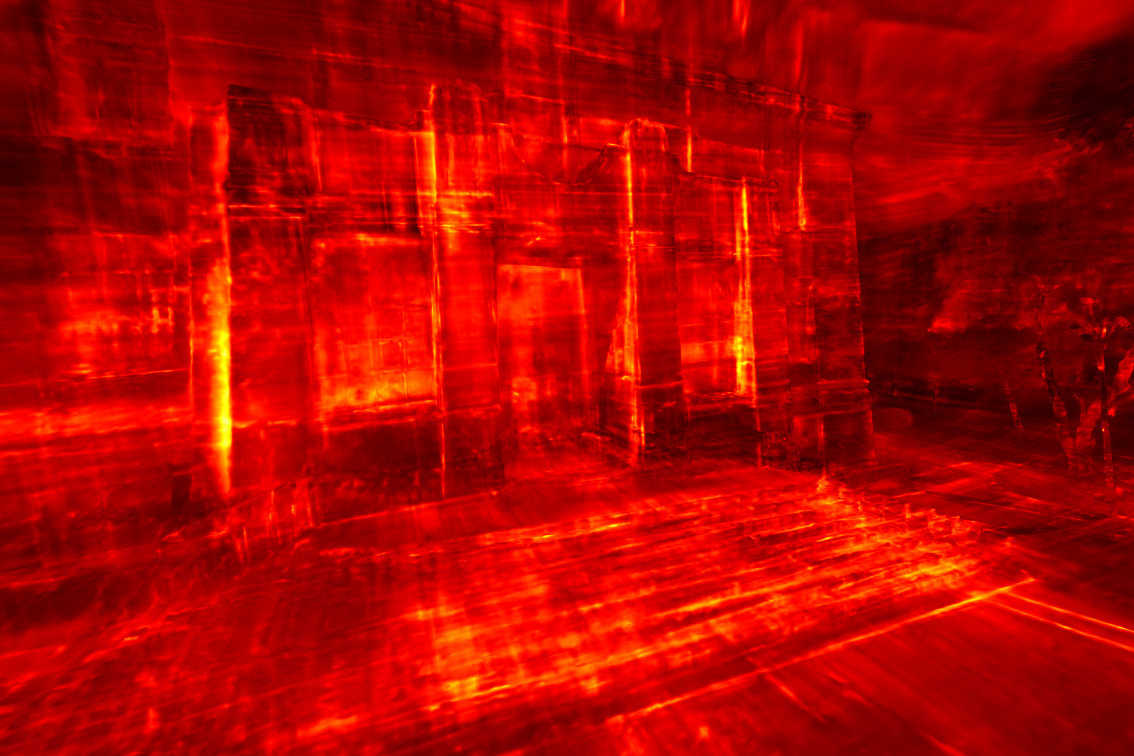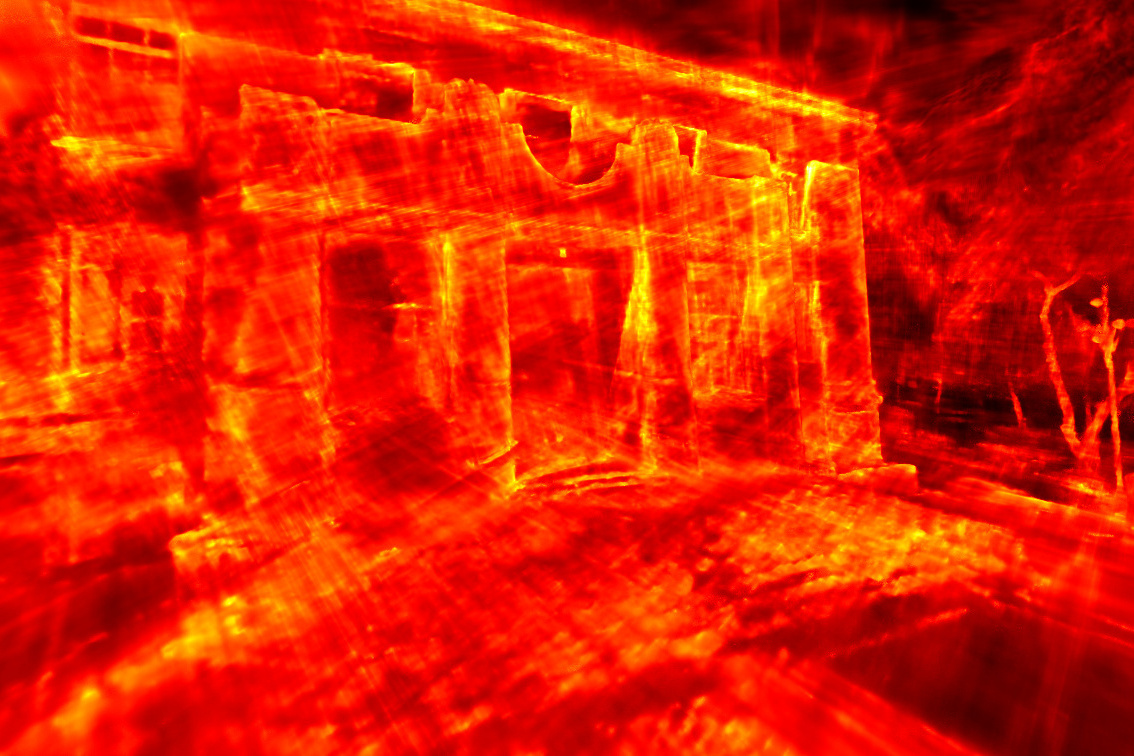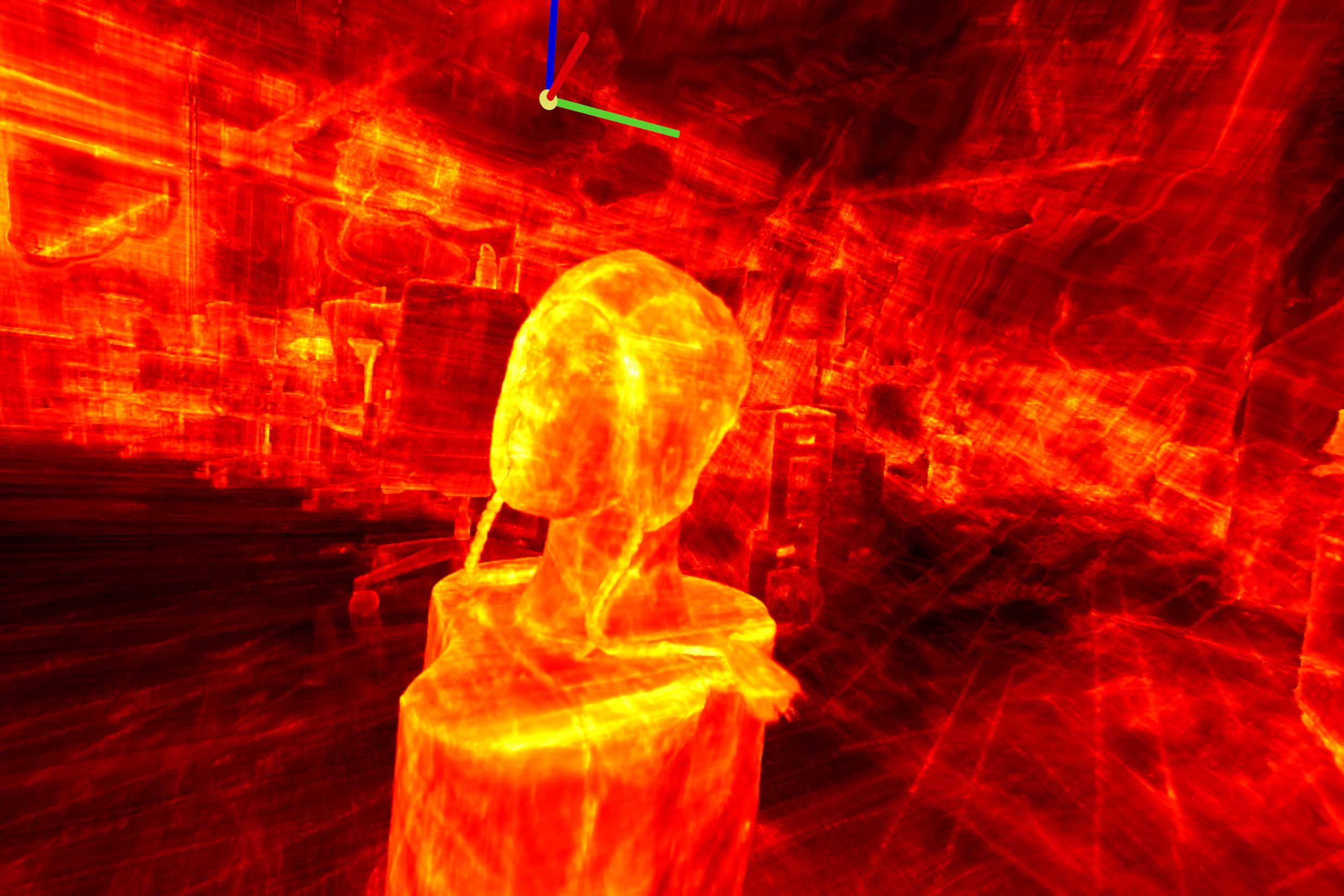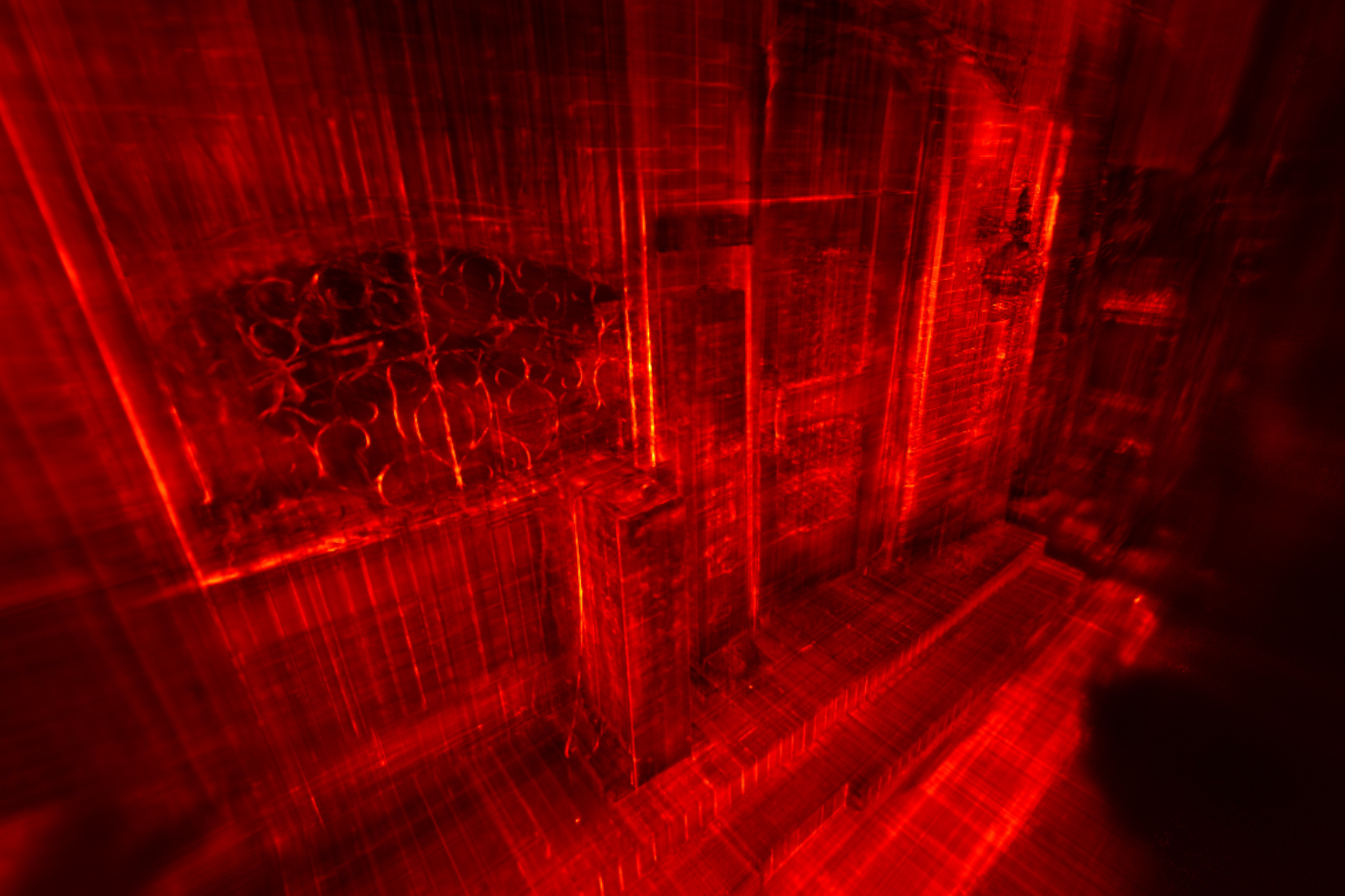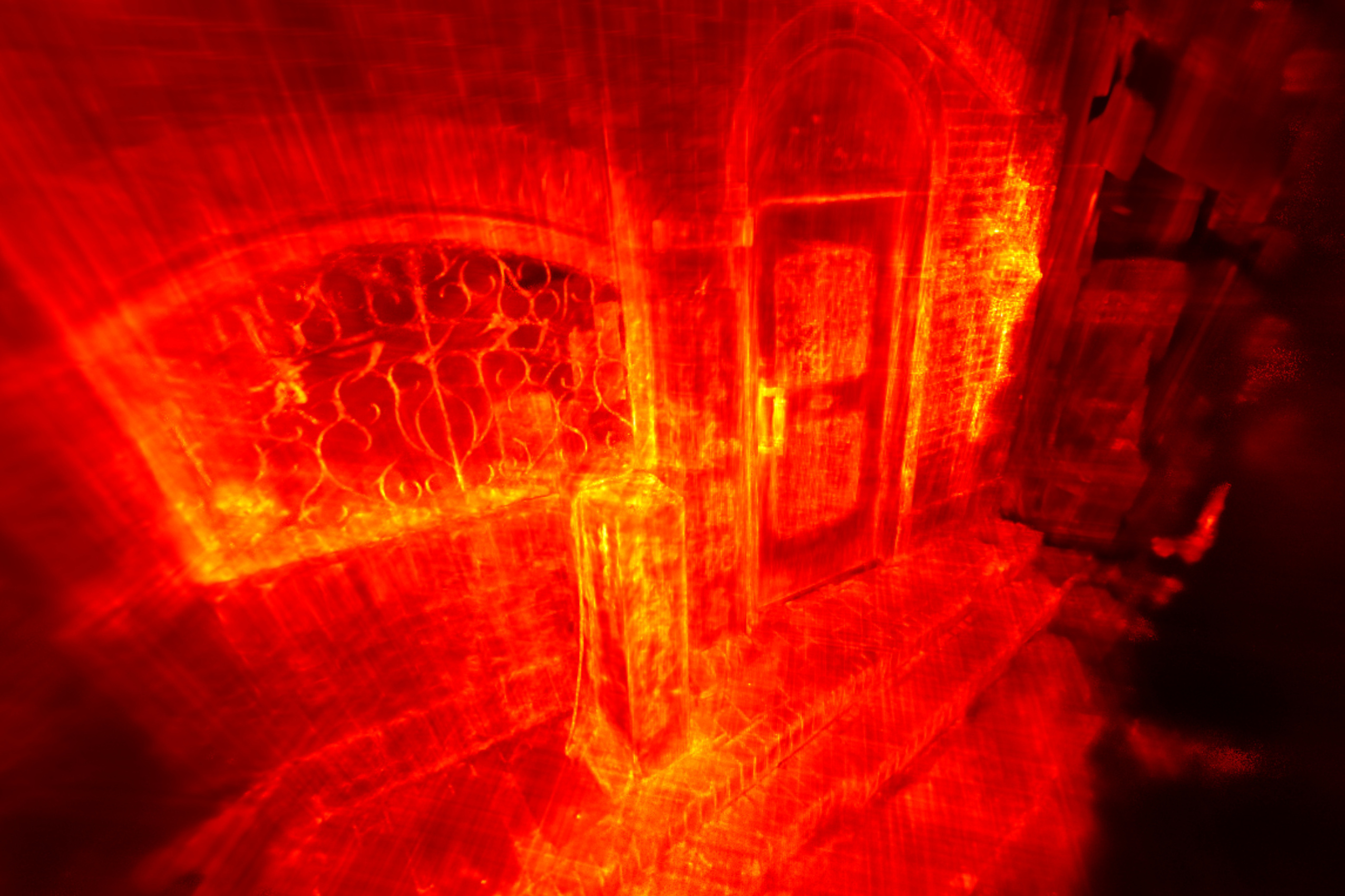From mapping latent feature vectors to RGB via a principal component analysis, we observe a more expressive and interpretable feature volume: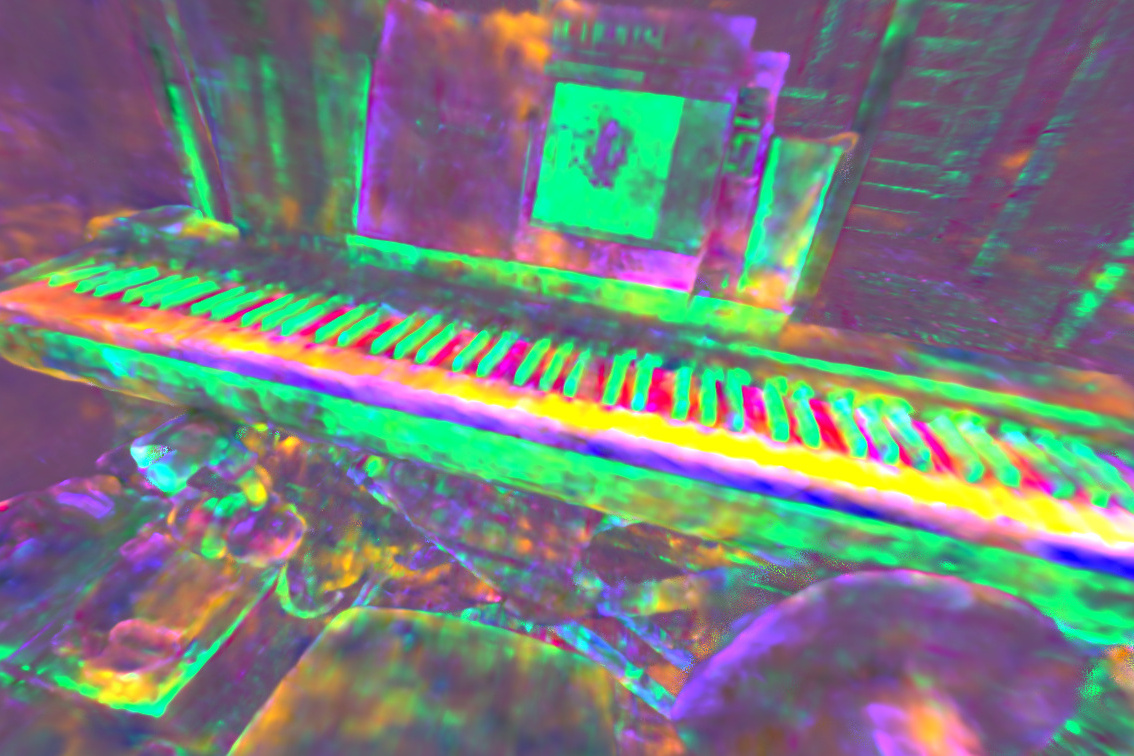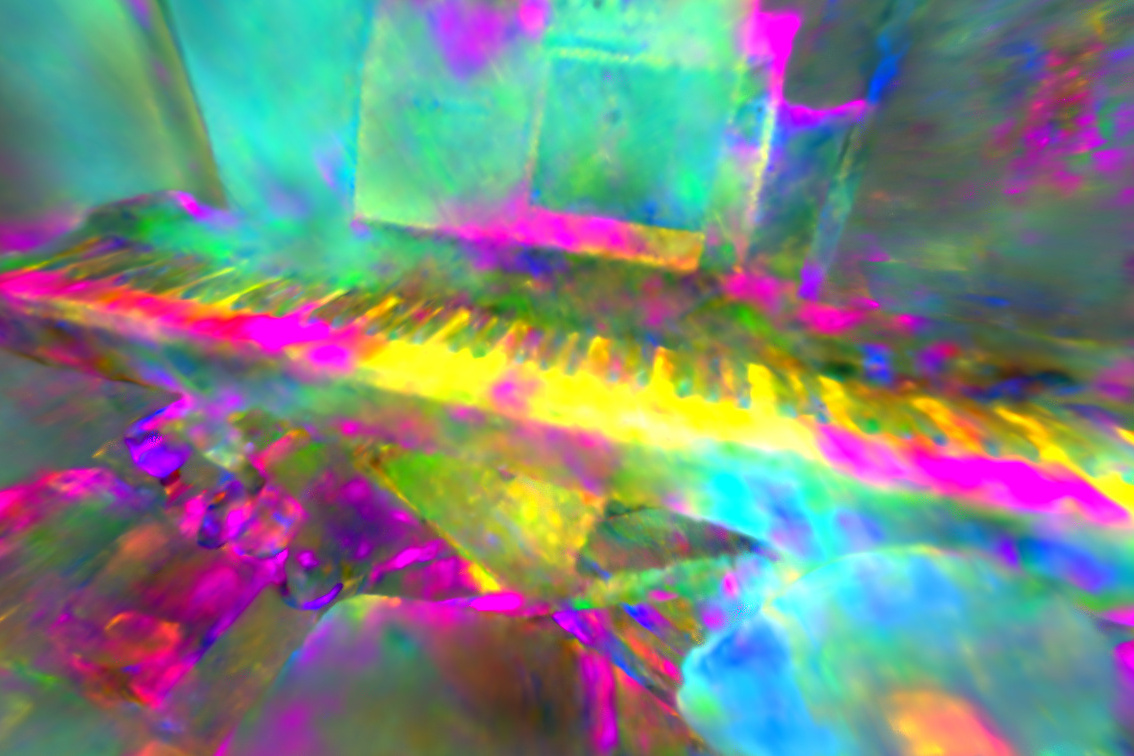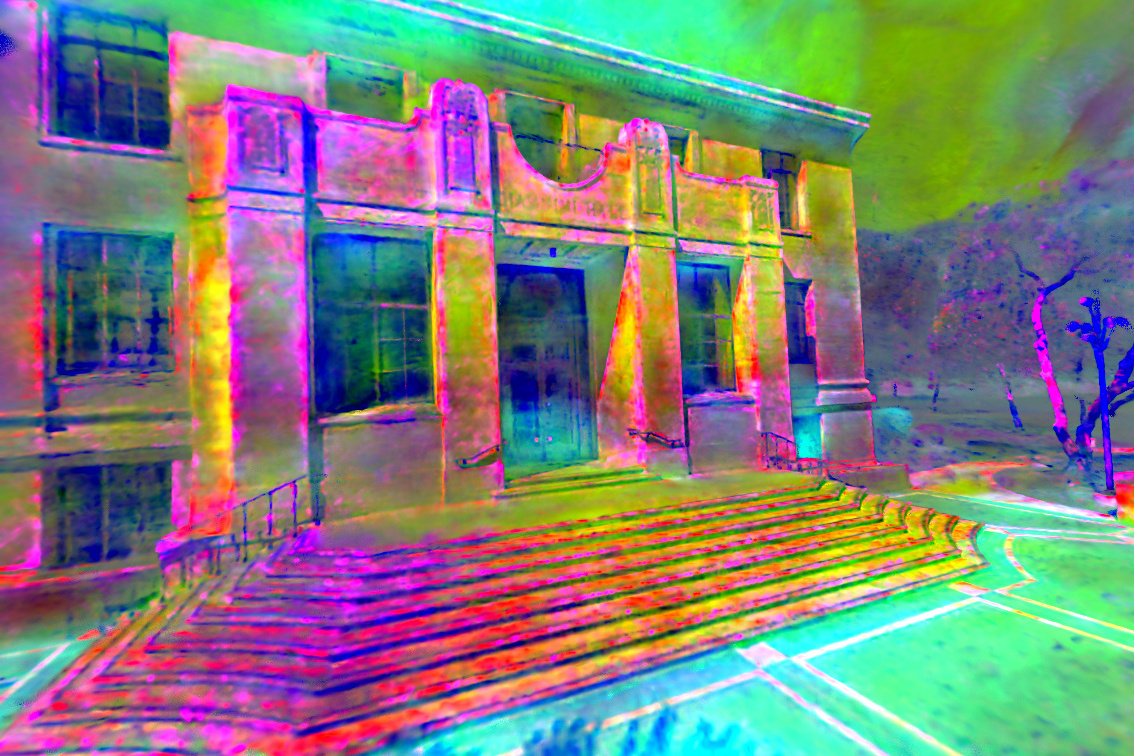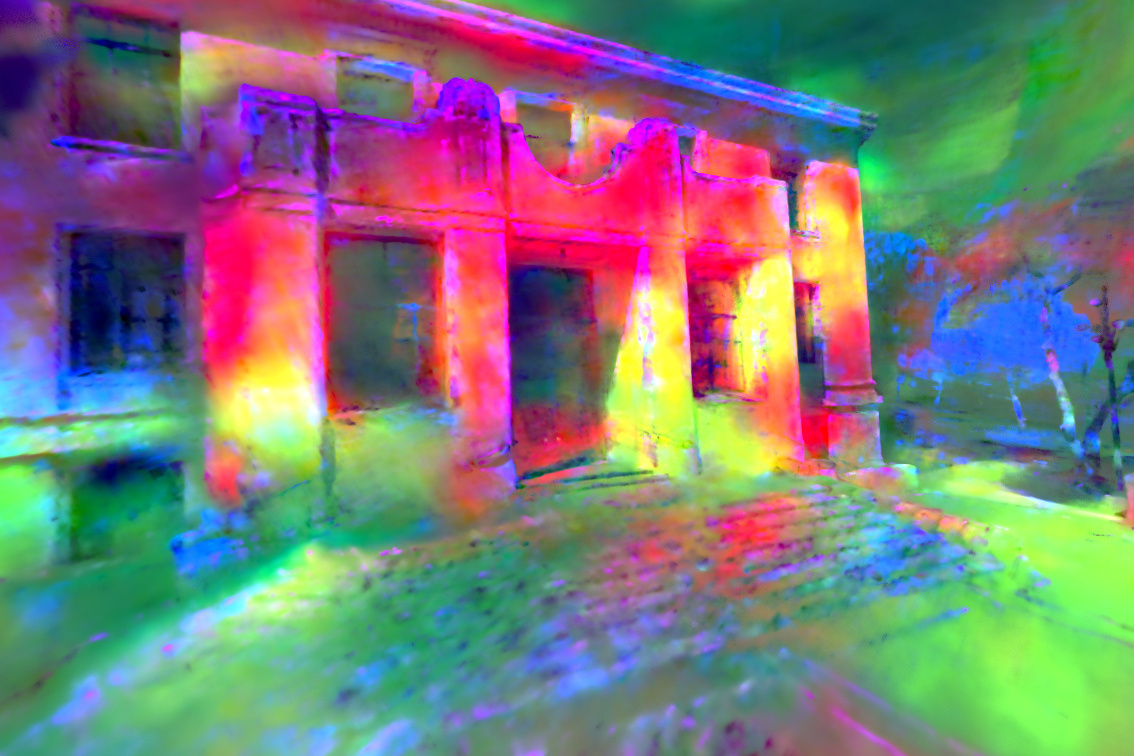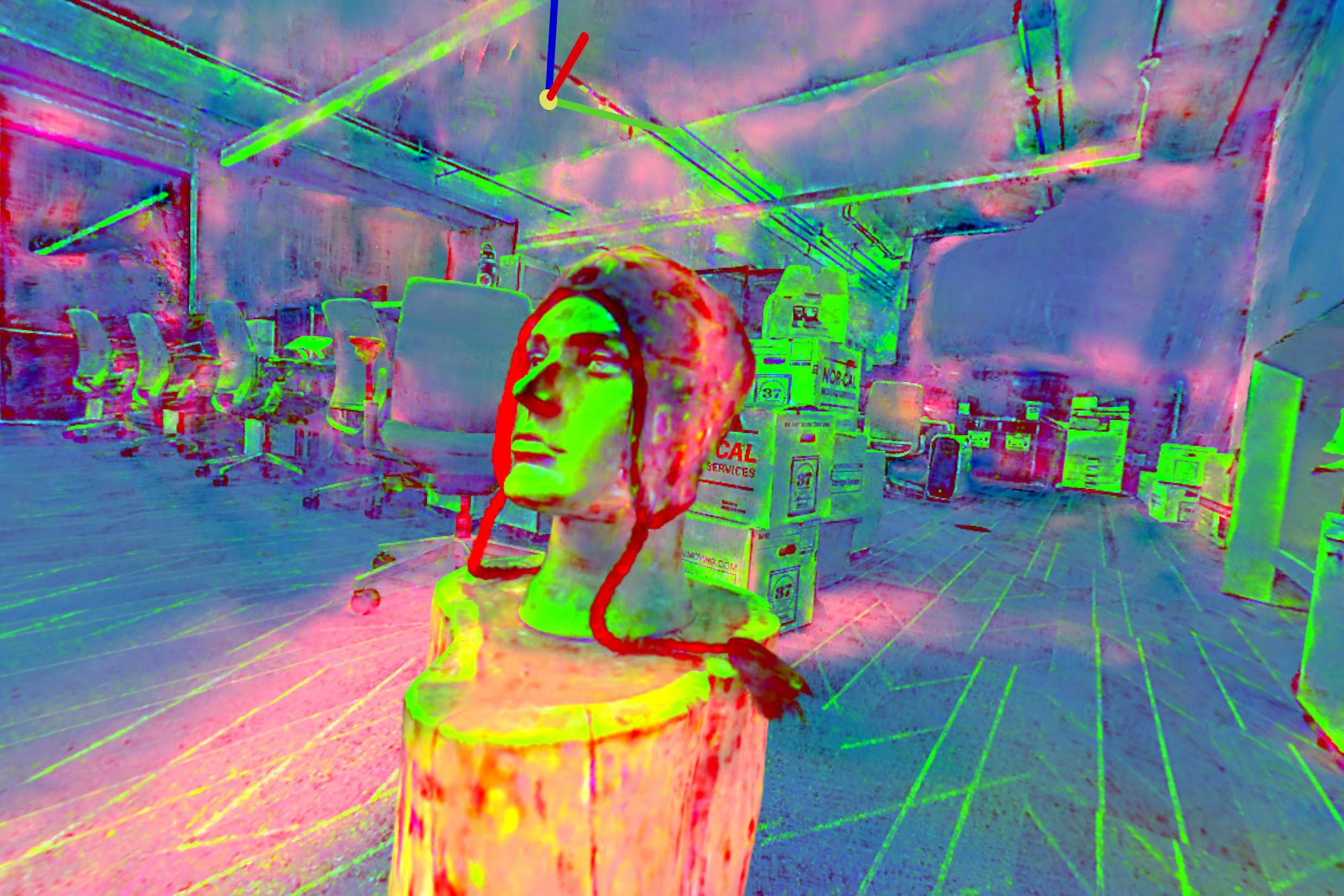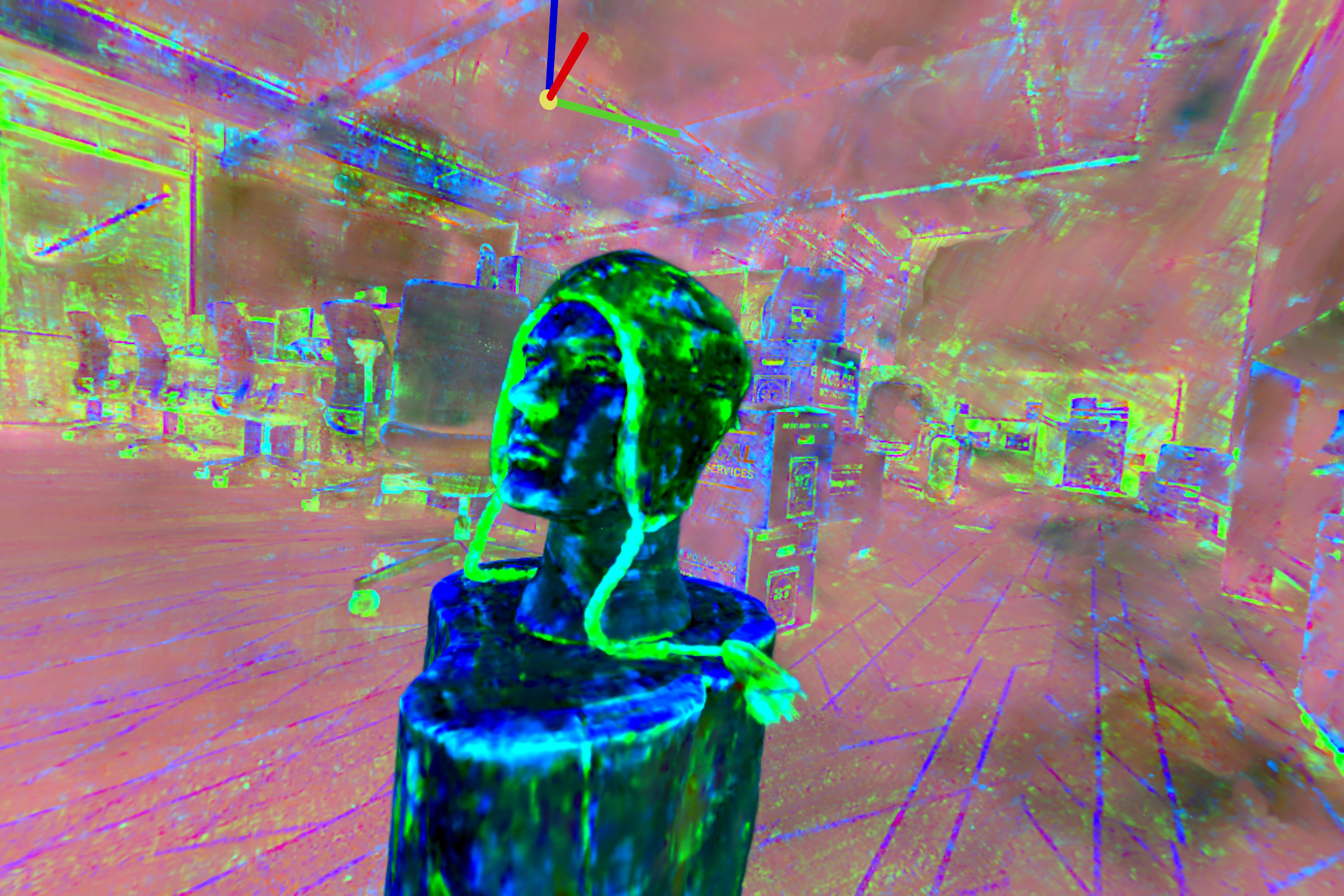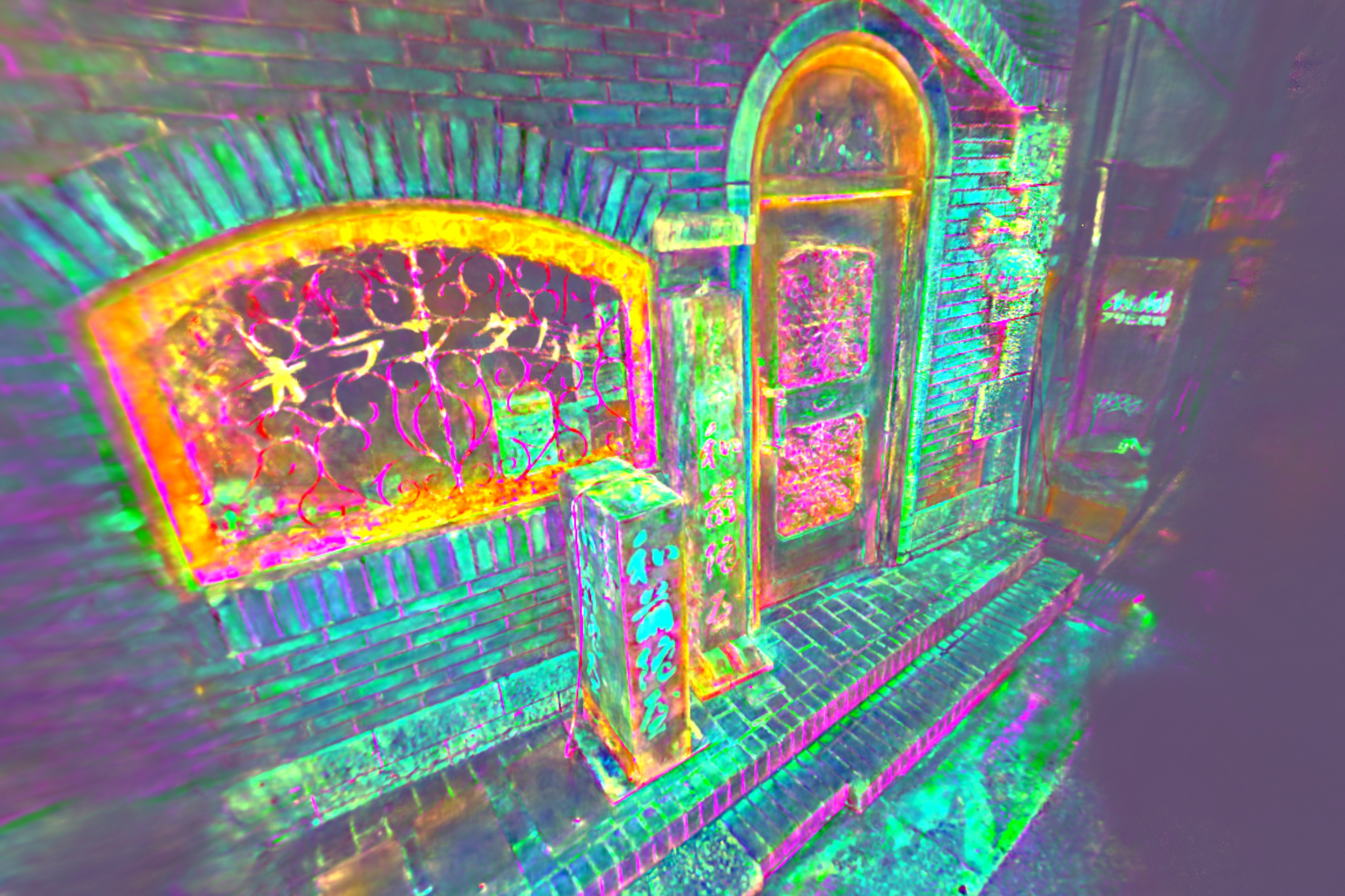## Motivation

Factored feature volumes offer advantages in compactness, efficiency, and interpretability, but biases are introduced by an implicit frame of representation.

The drawbacks of this are already apparent in 2D. A 2D factored grid is simply a low-rank matrix decomposition, whose compactness can be undermined by a simple rotation!

$\ \ \approx \newcommand{\w}{\color{lightgray}0} \newcommand{\b}{1} \Large \hspace{0.05em} \begin{bmatrix} \w & \w & \w & \w & \w \\ \w & \b & \b & \b & \w \\ \w & \b & \b & \b & \w \\ \w & \b & \b & \b & \w \\ \w & \w & \w & \w & \w \\ \end{bmatrix} \hspace{0.05em} \normalsize \approx \footnotesize \begin{bmatrix} \w & \w & \w & \w & \w & \w & \w & \w & \w \\ \w & \w & \w & \w & \w & \w & \w & \w & \w \\ \w & \w & \b & \b & \b & \b & \b & \w & \w \\ \w & \w & \b & \b & \b & \b & \b & \w & \w \\ \w & \w & \b & \b & \b & \b & \b & \w & \w \\ \w & \w & \b & \b & \b & \b & \b & \w & \w \\ \w & \w & \b & \b & \b & \b & \b & \w & \w \\ \w & \w & \w & \w & \w & \w & \w & \w & \w \\ \w & \w & \w & \w & \w & \w & \w & \w & \w \\ \end{bmatrix}$
rank 1 can capture infinite detail
$\ \ \approx \newcommand{\w}{\color{lightgray}0} \newcommand{\b}{1} \begin{bmatrix} \w & \w & \w & \w & \w & \w & \w \\ \w & \w & \w & \b & \w & \w & \w \\ \w & \w & \b & \b & \b & \w & \w \\ \w & \b & \b & \b & \b & \b & \w \\ \w & \w & \b & \b & \b & \w & \w \\ \w & \w & \w & \b & \w & \w & \w \\ \w & \w & \w & \w & \w & \w & \w \\ \end{bmatrix} \normalsize \approx \footnotesize \begin{bmatrix} \w & \w & \w & \w & \w & \w & \w & \w & \w \\ \w & \w & \w & \w & \b & \w & \w & \w & \w \\ \w & \w & \w & \b & \b & \b & \w & \w & \w \\ \w & \w & \b & \b & \b & \b & \b & \w & \w \\ \w & \b & \b & \b & \b & \b & \b & \b & \w \\ \w & \w & \b & \b & \b & \b & \b & \w & \w \\ \w & \w & \w & \b & \b & \b & \w & \w & \w \\ \w & \w & \w & \w & \b & \w & \w & \w & \w \\ \w & \w & \w & \w & \w & \w & \w & \w & \w \\ \end{bmatrix}$
rank grows with desired detail, despite equivalent information

TILTED overcomes this limitation of low-rank grids by jointly optimizing for canonical factors of the scene appearance and domain transformations that capture viewpoint and pose.

## Theoretical Grounding

Transformation optimization with a fixed representation (à la image registration) is a challenging problem that is prone to local minima; simultaneously learning the representation introduces further headaches. We develop the theoretical foundations for this methodology by proving that optimization recovers the scene appearance and transformation parameters in the 2D square example above. Concretely, we study an infinite-dimensional version of the optimization problem

$$\min_{\boldsymbol{U} \in \mathbb{R}^{n \times k},\, \phi \in [0, 2\pi]}\, \frac{1}{2} \left\| \boldsymbol{X}_{\diamond} - (\boldsymbol{U} \boldsymbol{U}^*) \circ \boldsymbol{\tau}_{\phi} \right\|_2^2,$$

and establish that an alternating minimization approach converges linearly to the square's true apperance and pose parameters, despite significant nonconvexity in the objective landscape.With an MLP decoder and regularization, our experiments extend this paradigm to more complex signals: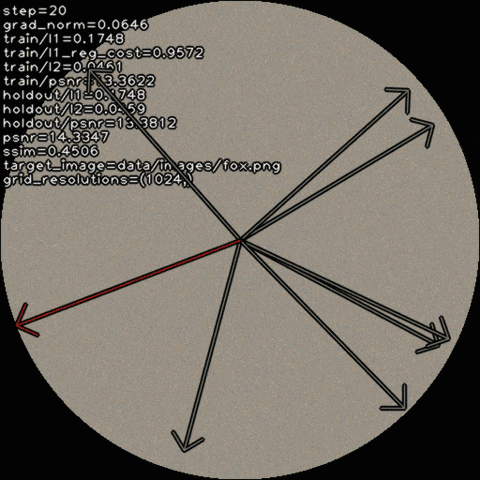Our analyses uncover a simple conceptual principle underlying the success of our method in idealized conditions: incremental improvements to representation promote incremental improvements to alignment, and vice versa, due to the constrained capacity of factored feature volumes. These capacity constraints obtain even when the rank of the grid is large, as a consequence of implicit regularization in matrix factorization problems towards low-rank solutions.

## Acknowledgements

This material is based upon work supported by the National Science Foundation Graduate Research Fellowship Program under Grant DGE 2146752, the ONR grant N00014-22-1-2102, and the joint Simons Foundation-NSF DMS grant 2031899.